Publicité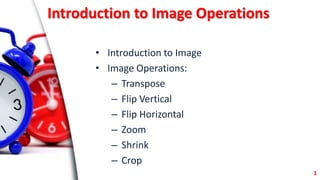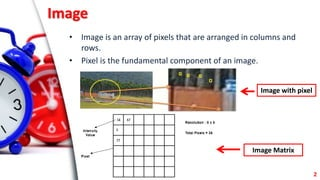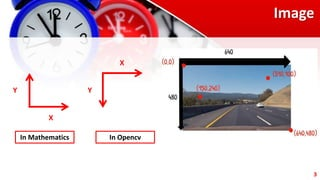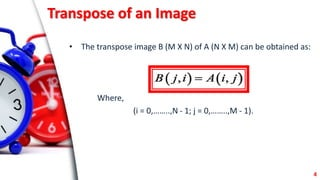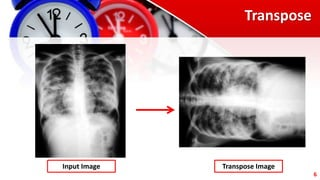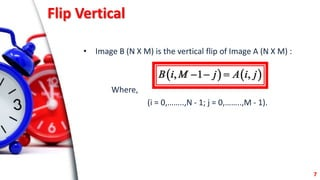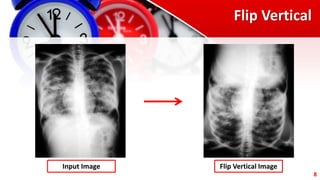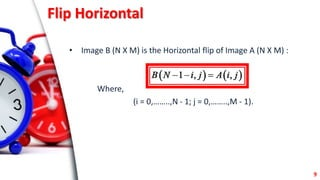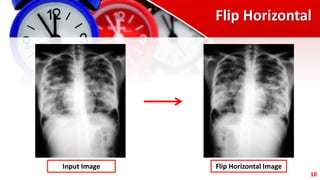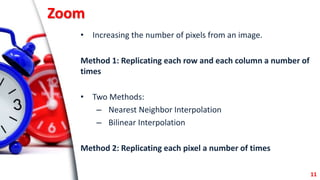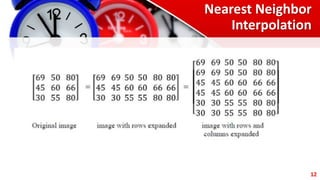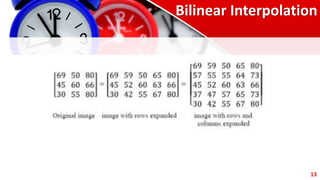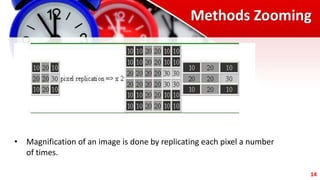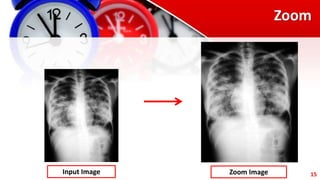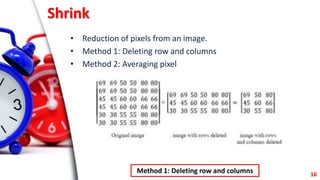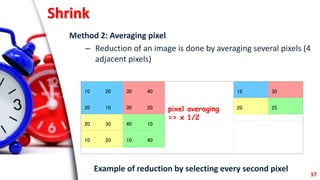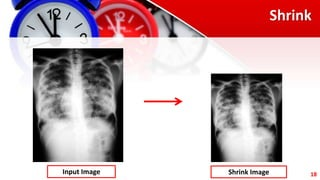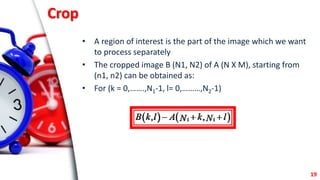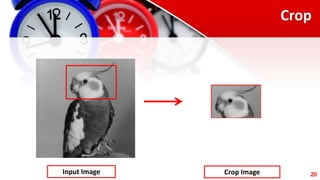1 sur 22
Publicité

### Day-1.pptx

1. Introduction to Image Operations Prof. Dr. Jharna Majumdar Prof. of Eminence, CUTM, BBSR Ms. Sasmita Kumari Nayak Mr. Nilamadhab Dash CSE, CUTM, BBSR
2. Introduction to Image Operations • Introduction to Image • Image Operations: – Transpose – Flip Vertical – Flip Horizontal – Zoom – Shrink – Crop 1
3. Image • Image is an array of pixels that are arranged in columns and rows. • Pixel is the fundamental component of an image. 2 Image Matrix Image with pixel
4. Image X Y X Y In Mathematics In Opencv 3
5. Transpose of an Image • The transpose image B (M X N) of A (N X M) can be obtained as: Where, (i = 0,……..,N - 1; j = 0,……..,M - 1).     , , B j i A i j  4
6. Transpose Input Image Transpose Image 5
7. Transpose Input Image Transpose Image 6
8. Flip Vertical • Image B (N X M) is the vertical flip of Image A (N X M) : Where, (i = 0,……..,N - 1; j = 0,……..,M - 1).     , 1 , B i M j A i j    7
9. Flip Vertical Input Image Flip Vertical Image 8
10. Flip Horizontal • Image B (N X M) is the Horizontal flip of Image A (N X M) : Where, (i = 0,……..,N - 1; j = 0,……..,M - 1).     1 , , B N i j A i j    9
11. Flip Horizontal Input Image Flip Horizontal Image 10
12. Zoom • Increasing the number of pixels from an image. Method 1: Replicating each row and each column a number of times • Two Methods: – Nearest Neighbor Interpolation – Bilinear Interpolation Method 2: Replicating each pixel a number of times 11
13. Nearest Neighbor Interpolation 12
14. Bilinear Interpolation 13
15. Methods Zooming 14 • Magnification of an image is done by replicating each pixel a number of times.
16. Zoom Input Image Zoom Image 15
17. Shrink • Reduction of pixels from an image. • Method 1: Deleting row and columns • Method 2: Averaging pixel 16 Method 1: Deleting row and columns
18. Shrink Method 2: Averaging pixel – Reduction of an image is done by averaging several pixels (4 adjacent pixels) 10 20 30 40 20 10 30 20 20 30 40 10 10 20 10 40 pixel averaging => x 1/2 15 30 20 25 Example of reduction by selecting every second pixel 17
19. Shrink Input Image Shrink Image 18
20. Crop • A region of interest is the part of the image which we want to process separately • The cropped image B (N1, N2) of A (N X M), starting from (n1, n2) can be obtained as: • For (k = 0,…….,N1-1, l= 0,………,N2-1)     1 1 , , B k l A k l N N    19
21. Crop Input Image Crop Image 20
Publicité